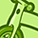# QlikView App Dev

Discussion Board for collaboration related to QlikView App Development.

Announcements
Join “Do More With Qlik” with Mike Tarallo to get a first-hand look at the New Authoring Experience, June 29th: REGISTER HERE
cancel
Showing results for
Did you mean:Partner

## Static Aggregation - ignoring filters

Hi

I have a straight table that i am trying to create where i need to fetch the number of days where the [lot numbers] executed were within the range - lot min & lot max targets. i do get the right number and fine.. but i would want the chart to only show for this month only and should not change when i pick any other filters / dates/months / city etc...completely ignore all selections

=

Sum ({1} Aggr(

if(

COUNT({1<

[Calendar Month] ={"=Month('\$(v_RptDate)')"}>} Distinct([Lot Number])) >= [Lot Min Target] AND

COUNT({1<

[Calendar Month] ={"=Month('\$(v_RptDate)')"}>} Distinct([Lot Number])) <= [Lot Max Target]

, COUNT({1<[Calendar Month] ={"=Month('\$(v_RptDate)')"}>} DISTINCT(CalendarDate)),0)

,CalendarDate, Location_City, Prtd_Type))

CalendarDate / City / Product type are dimensions.

appreciate any comment/feedback/help.

2 RepliesMVP

So based on what I understand, the expression above is working for you, you just need to restrict it in a way that it doesn't change based on any selections, right? Try this:

=Sum({1} Aggr(If(

Count({1<[Calendar Month] ={"=Month('\$(v_RptDate)')"}>} Distinct [Lot Number]) >= Only({1}[Lot Min Target])

and

Count({1<[Calendar Month] ={"=Month('\$(v_RptDate)')"}>} Distinct [Lot Number]) <= Only({1}[Lot Max Target]), Count({1<[Calendar Month] ={"=Month('\$(v_RptDate)')"}>} DISTINCTCalendarDate), 0), CalendarDate, Location_City, Prtd_Type))

The only other thing I would check is if the variable v_RptDate has an expression where you would need to ignore selection using {1}.Partner
Author